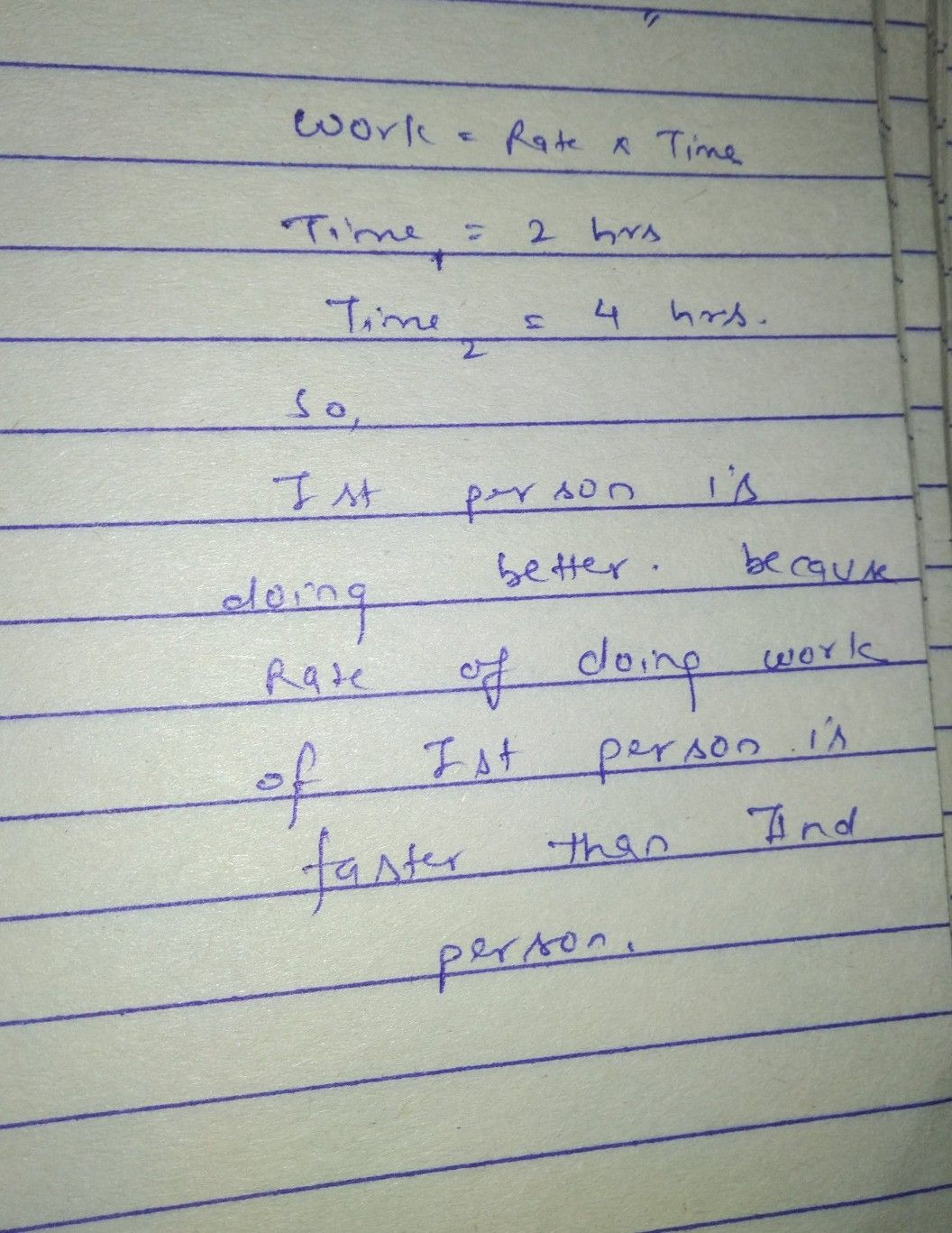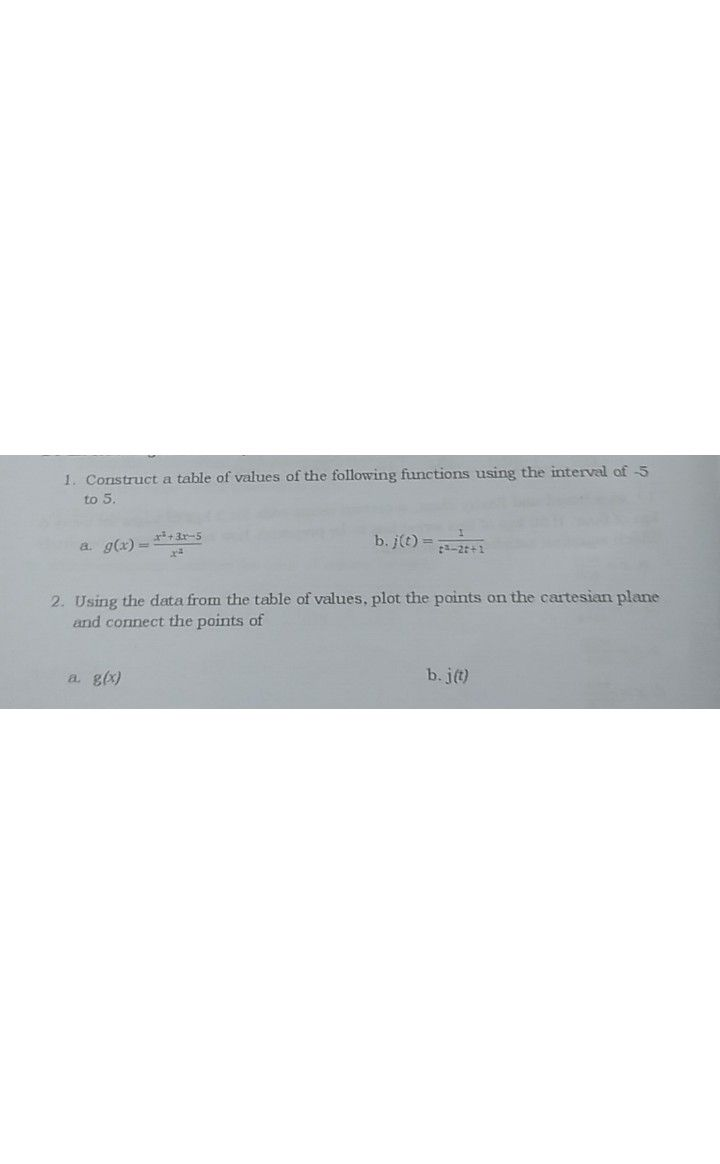Symbol
Problem$An$ application of rational functions may involve the number of $\square$ persons $Ta0$ $can$ do a task in a certain amount of time. We can handle these applications involving work in a manner similar to the method $5e$ used to solve distance, speed, and tìme $problems$ $Woxk=$ $R$ Rate $X$ Time. Suppose you can finish a report $1\pi$ $2$ hours. Your classmate can finish the same report in $4$ hours. How long will $it$ take to finish the report if both of you work together? $BIe$ $haV8$ a saying that $1\pi 0$ $0$ heads are better than $one^{7}$ would you rather work alone or with a team? Why?
10th-13th grade
Other
Search count: 105
SolutionQanda teacher - sonammam
if you have any further queries regarding this question please feel free to ask thank you so muchStudent
Thank you